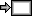Using the TI-84 Plus Video Series Premieres

To mark the publication of Using the TI-84 Plus, Second Edition, I'm happy to announce a new video series, "Using the TI-84 Plus CE". Based on the chapters in Using the TI-84 Plus, each video will introduce key calculator skills that students need to know to use their calculators efficiently for math, science, and even programming. Throughout the video series, you'll learn:
1. Basic calculator skills, arithmetic, simple trig (Chapter 2)
2. Basic graphing (Chapter 3)
3. Using variables, matrices, and lists (Chapter 4)
4. Parametric, polar, and sequence graphing (Chapter 5)
5. Precalculus: imaginary numbers and trig (Chapter 6)
6. Calculus: derivatives and integrals (Chapter 7)
7. An introduction to statistics and probability (chapters 8 & 9)
8. Financial tools (Chapter 10)
9. Programming (Chapter 11)
Each video will give a brief introduction to vital calculator skills, then direct you to the relevant section(s) in the book for more information. I hope you enjoy Part 1 (linked below), and I'd welcome feedback, comments, and suggestions.

View the SeriesUsing the TI-84 Plus CE video seriesUsing the TI-84 Plus, Second Edition